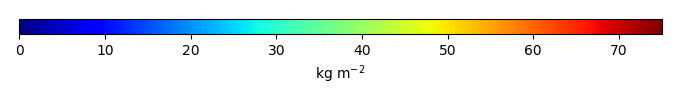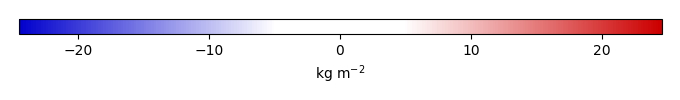# Mean State

Period Mean (original grids) [Pg]
Model Period Mean (intersection) [Pg]
Model Period Mean (complement) [Pg]
Benchmark Period Mean (intersection) [Pg]
Benchmark Period Mean (complement) [Pg]
Bias [kg m-2]
Bias Score 
Spatial Distribution Score 
Overall Score 
Benchmark [-] 34.1
CLM5PHSOFF [-] 33.5 32.8 1.04 33.8 0.315 -0.225 0.707 0.943 0.825
CLM5PHSON [-] 30.5 30.9 0.269 33.8 0.315 -0.226 0.729 0.948 0.839
Period Mean (original grids) [Pg]
Model Period Mean (intersection) [Pg]
Model Period Mean (complement) [Pg]
Benchmark Period Mean (intersection) [Pg]
Benchmark Period Mean (complement) [Pg]
Bias [kg m-2]
Bias Score 
Spatial Distribution Score 
Overall Score 
Benchmark [-] 44.8
CLM5PHSOFF [-] 14.4 13.7 0.0504 44.4 0.478 -4.51 0.498 0.639 0.569
CLM5PHSON [-] 15.3 14.4 0.0241 44.4 0.478 -4.37 0.502 0.776 0.639
Period Mean (original grids) [Pg]
Model Period Mean (intersection) [Pg]
Model Period Mean (complement) [Pg]
Benchmark Period Mean (intersection) [Pg]
Benchmark Period Mean (complement) [Pg]
Bias [kg m-2]
Bias Score 
Spatial Distribution Score 
Overall Score 
Benchmark [-] 60.1
CLM5PHSOFF [-] 31.2 27.7 2.83 59.3 0.762 -3.34 0.542 0.843 0.693
CLM5PHSON [-] 28.7 27.8 0.749 59.3 0.762 -2.94 0.548 0.836 0.692
Period Mean (original grids) [Pg]
Model Period Mean (intersection) [Pg]
Model Period Mean (complement) [Pg]
Benchmark Period Mean (intersection) [Pg]
Benchmark Period Mean (complement) [Pg]
Bias [kg m-2]
Bias Score 
Spatial Distribution Score 
Overall Score 
Benchmark [-] 11.1
CLM5PHSOFF [-] 67.2 9.67 57.7 10.3 0.803 -1.42 0.406 0.309 0.357
CLM5PHSON [-] 101. 9.11 91.2 10.3 0.803 7.12 0.427 0.186 0.307
Period Mean (original grids) [Pg]
Model Period Mean (intersection) [Pg]
Model Period Mean (complement) [Pg]
Benchmark Period Mean (intersection) [Pg]
Benchmark Period Mean (complement) [Pg]
Bias [kg m-2]
Bias Score 
Spatial Distribution Score 
Overall Score 
Benchmark [-] 55.7
CLM5PHSOFF [-] 41.7 33.9 6.36 50.7 4.99 -4.94 0.682 0.304 0.493
CLM5PHSON [-] 20.6 19.1 1.31 50.7 4.99 -6.12 0.649 0.274 0.461
Period Mean (original grids) [Pg]
Model Period Mean (intersection) [Pg]
Model Period Mean (complement) [Pg]
Benchmark Period Mean (intersection) [Pg]
Benchmark Period Mean (complement) [Pg]
Bias [kg m-2]
Bias Score 
Spatial Distribution Score 
Overall Score 
Benchmark [-] 65.6
CLM5PHSOFF [-] 74.8 37.0 35.8 65.2 0.461 -6.70 0.620 0.861 0.741
CLM5PHSON [-] 133. 59.0 71.0 65.2 0.461 -1.06 0.711 0.837 0.774
Period Mean (original grids) [Pg]
Model Period Mean (intersection) [Pg]
Model Period Mean (complement) [Pg]
Benchmark Period Mean (intersection) [Pg]
Benchmark Period Mean (complement) [Pg]
Bias [kg m-2]
Bias Score 
Spatial Distribution Score 
Overall Score 
Benchmark [-] 143.
CLM5PHSOFF [-] 63.0 59.5 4.76 141. 2.09 -4.68 0.549 0.842 0.695
CLM5PHSON [-] 91.8 88.3 5.88 141. 2.09 -2.73 0.621 0.909 0.765
Period Mean (original grids) [Pg]
Model Period Mean (intersection) [Pg]
Model Period Mean (complement) [Pg]
Benchmark Period Mean (intersection) [Pg]
Benchmark Period Mean (complement) [Pg]
Bias [kg m-2]
Bias Score 
Spatial Distribution Score 
Overall Score 
Benchmark [-] 58.4
CLM5PHSOFF [-] 36.8 32.2 3.01 56.8 1.57 -3.42 0.603 0.824 0.713
CLM5PHSON [-] 33.0 31.1 0.855 56.8 1.57 -2.67 0.656 0.862 0.759
Period Mean (original grids) [Pg]
Model Period Mean (intersection) [Pg]
Model Period Mean (complement) [Pg]
Benchmark Period Mean (intersection) [Pg]
Benchmark Period Mean (complement) [Pg]
Bias [kg m-2]
Bias Score 
Spatial Distribution Score 
Overall Score 
Benchmark [-] 7.78
CLM5PHSOFF [-] 87.0 9.96 78.0 7.36 0.414 6.55 0.447 0.350 0.398
CLM5PHSON [-] 119. 9.41 111. 7.36 0.414 11.0 0.450 0.207 0.328
Period Mean (original grids) [Pg]
Model Period Mean (intersection) [Pg]
Model Period Mean (complement) [Pg]
Benchmark Period Mean (intersection) [Pg]
Benchmark Period Mean (complement) [Pg]
Bias [kg m-2]
Bias Score 
Spatial Distribution Score 
Overall Score 
Benchmark [-] 58.9
CLM5PHSOFF [-] 31.3 26.4 4.46 57.4 1.53 -3.60 0.531 0.626 0.579
CLM5PHSON [-] 27.6 25.8 1.60 57.4 1.53 -2.75 0.541 0.473 0.507
Period Mean (original grids) [Pg]
Model Period Mean (intersection) [Pg]
Model Period Mean (complement) [Pg]
Benchmark Period Mean (intersection) [Pg]
Benchmark Period Mean (complement) [Pg]
Bias [kg m-2]
Bias Score 
Spatial Distribution Score 
Overall Score 
Benchmark [-] 61.8
CLM5PHSOFF [-] 39.5 33.0 5.63 60.2 1.58 -4.92 0.629 0.840 0.735
CLM5PHSON [-] 44.2 39.3 4.23 60.2 1.58 -2.36 0.718 0.846 0.782
Period Mean (original grids) [Pg]
Model Period Mean (intersection) [Pg]
Model Period Mean (complement) [Pg]
Benchmark Period Mean (intersection) [Pg]
Benchmark Period Mean (complement) [Pg]
Bias [kg m-2]
Bias Score 
Spatial Distribution Score 
Overall Score 
Benchmark [-] 1.09e+03
CLM5PHSOFF [-] 886. 543. 342. 1.03e+03 55.6 -4.07 0.576 0.476 0.526
CLM5PHSON [-] 1.06e+03 608. 446. 1.03e+03 55.6 -2.80 0.621 0.752 0.686
Period Mean (original grids) [Pg]
Model Period Mean (intersection) [Pg]
Model Period Mean (complement) [Pg]
Benchmark Period Mean (intersection) [Pg]
Benchmark Period Mean (complement) [Pg]
Bias [kg m-2]
Bias Score 
Spatial Distribution Score 
Overall Score 
Benchmark [-] 64.3
CLM5PHSOFF [-] 7.36 6.25 1.08 63.6 0.726 -3.51 0.410 0.615 0.513
CLM5PHSON [-] 14.0 13.8 0.386 63.6 0.726 -2.91 0.482 0.820 0.651
Period Mean (original grids) [Pg]
Model Period Mean (intersection) [Pg]
Model Period Mean (complement) [Pg]
Benchmark Period Mean (intersection) [Pg]
Benchmark Period Mean (complement) [Pg]
Bias [kg m-2]
Bias Score 
Spatial Distribution Score 
Overall Score 
Benchmark [-] 13.3
CLM5PHSOFF [-] 73.1 10.4 62.2 12.1 1.24 -1.60 0.485 0.309 0.397
CLM5PHSON [-] 123. 8.60 114. 12.1 1.24 -0.123 0.473 0.574 0.524
Period Mean (original grids) [Pg]
Model Period Mean (intersection) [Pg]
Model Period Mean (complement) [Pg]
Benchmark Period Mean (intersection) [Pg]
Benchmark Period Mean (complement) [Pg]
Bias [kg m-2]
Bias Score 
Spatial Distribution Score 
Overall Score 
Benchmark [-] 42.5
CLM5PHSOFF [-] 23.2 19.6 2.87 40.2 2.26 -6.41 0.560 0.676 0.618
CLM5PHSON [-] 16.9 15.5 0.942 40.2 2.26 -5.56 0.571 0.733 0.652
Period Mean (original grids) [Pg]
Model Period Mean (intersection) [Pg]
Model Period Mean (complement) [Pg]
Benchmark Period Mean (intersection) [Pg]
Benchmark Period Mean (complement) [Pg]
Bias [kg m-2]
Bias Score 
Spatial Distribution Score 
Overall Score 
Benchmark [-] 45.3
CLM5PHSOFF [-] 20.3 18.7 0.873 44.4 0.888 -6.20 0.575 0.576 0.576
CLM5PHSON [-] 32.2 31.2 0.326 44.4 0.888 -2.77 0.722 0.773 0.747
Period Mean (original grids) [Pg]
Model Period Mean (intersection) [Pg]
Model Period Mean (complement) [Pg]
Benchmark Period Mean (intersection) [Pg]
Benchmark Period Mean (complement) [Pg]
Bias [kg m-2]
Bias Score 
Spatial Distribution Score 
Overall Score 
Benchmark [-] 51.3
CLM5PHSOFF [-] 45.7 24.0 22.1 49.6 1.76 -7.84 0.561 0.826 0.693
CLM5PHSON [-] 75.2 39.4 36.1 49.6 1.76 -2.45 0.632 0.880 0.756
Period Mean (original grids) [Pg]
Model Period Mean (intersection) [Pg]
Model Period Mean (complement) [Pg]
Benchmark Period Mean (intersection) [Pg]
Benchmark Period Mean (complement) [Pg]
Bias [kg m-2]
Bias Score 
Spatial Distribution Score 
Overall Score 
Benchmark [-] 34.3
CLM5PHSOFF [-] 7.06 7.21 0.410 34.0 0.216 -6.81 0.461 0.731 0.596
CLM5PHSON [-] 11.5 11.8 0.377 34.0 0.216 -5.39 0.539 0.874 0.706
Period Mean (original grids) [Pg]
Model Period Mean (intersection) [Pg]
Model Period Mean (complement) [Pg]
Benchmark Period Mean (intersection) [Pg]
Benchmark Period Mean (complement) [Pg]
Bias [kg m-2]
Bias Score 
Spatial Distribution Score 
Overall Score 
Benchmark [-] 101.
CLM5PHSOFF [-] 74.2 72.2 1.62 100. 0.811 -3.26 0.691 0.517 0.604
CLM5PHSON [-] 68.5 67.8 0.372 100. 0.811 -3.35 0.692 0.464 0.578
Period Mean (original grids) [Pg]
Model Period Mean (intersection) [Pg]
Model Period Mean (complement) [Pg]
Benchmark Period Mean (intersection) [Pg]
Benchmark Period Mean (complement) [Pg]
Bias [kg m-2]
Bias Score 
Spatial Distribution Score 
Overall Score 
Benchmark [-] 60.7
CLM5PHSOFF [-] 41.4 40.3 1.47 60.4 0.300 -2.91 0.646 0.759 0.702
CLM5PHSON [-] 47.2 47.5 0.479 60.4 0.300 -1.44 0.672 0.836 0.754
Period Mean (original grids) [Pg]
Model Period Mean (intersection) [Pg]
Model Period Mean (complement) [Pg]
Benchmark Period Mean (intersection) [Pg]
Benchmark Period Mean (complement) [Pg]
Bias [kg m-2]
Bias Score 
Spatial Distribution Score 
Overall Score 
Benchmark [-] 29.6
CLM5PHSOFF [-] 16.2 14.1 1.51 28.2 1.46 -4.22 0.525 0.544 0.534
CLM5PHSON [-] 15.7 14.8 0.272 28.2 1.46 -3.23 0.571 0.493 0.532

# Temporally integrated period mean

BENCHMARK MEANMODEL MEANBIASBIAS SCORESPATIAL TAYLOR DIAGRAMMODEL COLORS# Spatially integrated regional mean

MODEL COLORS# All Models

BenchmarkCLM5PHSOFFCLM5PHSON# Data Information

Approach: I just read the data from the original data file and flip the latitude arrange from original 90N->90S to 90S->90N.

Temporal resolution: yearly

General information: This product was derived from Harmonized World Soil Database (HWSD) v1.2 dataset.

Spatial resolution: 0.5x0.5 degree